### PAPER:

Wavelet bases made of piecewise polynomial functions: approximation theory, quadrature rules and applications  to integral kernel sparsification and image compression *

Lorella Fatone

Dipartimento di Matematica e Informatica, Università di Camerino, Via Madonna delle Carceri 9, 62032 Camerino, Italy. Ph. N. +39-0737-402558, Fax N. +39-0737-632525, e-mail: lorella.fatone@unicam.it

Maria Cristina Recchioni

Dipartimento di Scienze Sociali “D. Serrani”, Università Politecnica delle Marche, Piazza Martelli 8, 60121 Ancona, Italy. Ph. N. +39-071-2207066, Fax N. +39-071-2207150, e-mail: m.c.recchioni@univpm.it

Francesco Zirilli

Dipartimento di Matematica “G. Castelnuovo”, Università di Roma “La Sapienza”, Piazzale Aldo Moro 2, 00185 Roma, Italy. Ph. N. +39-06-49913282, Fax N. +39-06-44701007, e-mail: f.zirilli@caspur.it

1.   Abstract

2.    Introduction

3.    Examples of MATLAB Symbolic Math codes that compute the wavelet mother functions of the wavelet bases used in the numerical experiments

4.  Some numerical experiments: integral kernel sparsification, image compression and reconstruction

5.   References

1. Abstract

We present wavelet bases made of piecewise (low degree) polynomial functions with an (arbitrary) assigned number of vanishing moments. We study some of the properties of  these wavelet bases; in particular we consider their use in the approximation of functions and in numerical quadrature. We focus on two relevant applications: integral kernel sparsification  and digital image compression and reconstruction. In these application areas the use of the wavelet bases presented gives very satisfactory  results. This website contains auxiliary material and animations that help the understanding of the paper  and makes available to the interested users some software programs that generate the wavelet mother functions of the wavelet bases used to produce the numerical results presented.

The numerical experience reported in this website and in the paper   has been obtained using the computing resources of CASPUR (Roma, Italy) under grant: “Algoritmi di alte prestazioni per problemi di scattering acustico”. The support and sponsorship of CASPUR are gratefully acknowledged.

2.  Introduction

In the last few decades wavelets and wavelets techniques have generated much interest, both in  mathematical analysis as well as in signal processing and in many other application fields. The link between the mathematical analysis and signal processing approaches to the study of wavelets was given by Coifman, Mallat and Meyer (see , , , , , ) with the introduction of multiresolution analysis and  of the fast wavelet transform, and by Daubechies (see ) with the introduction of orthonormal bases of compactly supported wavelets.

Let A be an open subset of a real Euclidean space and let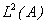be the Hilbert space of the square integrable (with respect to Lebesgue measure) real functions defined on A. In  when A is a sufficiently simple set (i.e. a parallelepiped in the examples considered), starting from the notion of multiresolution analysis, we construct wavelet bases ofwith an (arbitrary) assigned number of vanishing moments. In this website we restrict our attention to the case A=(0,1). The main feature of these wavelet bases is that they are made of piecewise polynomial functions of compact support; moreover the polynomials used are of low degree and generate bases with an arbitrarily high assigned number of vanishing moments. This fact makes possible to perform very efficiently some of the most common computations, such as, for example, differentiation and integration. However the lack of regularity of the piecewise polynomial functions used can create undesirable effects in the  points where the discontinuities occur when, for example, continuous functions are approximated with discontinuous functions. Note that the wavelet bases studied in , in general, make use of more than one wavelet mother function. Thanks to these properties these wavelet bases in several applications can outperform in actual computations the classical wavelet bases and, for example, in  we show that they have very good approximation and compression properties. The numerical results shown in Section 4 and in  corroborate these statements both from the qualitative and the quantitative point of view.

The wavelet bases introduced generalize the classical Haar's basis, that has only one vanishing moment and is made of piecewise constant functions (see, for example, ), and are a simple variation of the multi-wavelets bases introduced by Alpert in , . The results reported here and in  extend those reported in ,  and aim to show not only the theoretical relevance of these wavelet bases (also shown, for example, in , , , ) but also their effective applicability in real problems. In particular in  we study some properties of the  wavelet bases considered and the advantages of using some of them in simple circumstances. In particular, the orthogonality of the wavelets to the polynomials up to a given degree (i.e. the vanishing moments property) plays a crucial role in producing “sparsity” in the representation using these wavelet bases of functions, integral kernels, images and so on. These  wavelet bases as the wavelet bases used previously have good localization properties both in the spatial and in the frequency domains and can be used fruitfully in several classical problems of functional analysis. In particular in  we focus on the representation of a function in the wavelet bases and  we present some ad hoc quadrature formulae that can be used to compute efficiently the coefficients of the wavelet expansion of a function. We consider also  the use of these  wavelet bases in some applications, initially we focus on integral kernel sparsification. This is a relevant task, see for example , , since it makes possible, among other things, the approximation of some integral operators with sparse matrices allowing the approximation and the solution of the corresponding integral equations in very high dimensional subspaces at an affordable computational cost. In ,  and , for example, we exploit this property to solve some time dependent acoustic obstacle scattering problems involving realistic objects hit by waves of small wavelength when compared to the dimension of the objects. Let us note that these scattering problems are translated  mathematically in problems for the wave equation and that they are numerically challenging, moreover thanks to the use of the wavelet bases, when boundary integral methods or some of their variations are used, they can be approximated by sparse systems of linear equations in very high dimensional spaces (i.e. linear systems with millions of unknowns and equations).

Later on we focus on another important application of wavelets: digital image compression and reconstruction. In this framework, the basic idea is to distinguish between relevant and less relevant parts of the image details disregarding, if  necessary, the second ones. In particular we proceed as follows: a digital image is represented as a sequence of wavelet coefficients (wavelet transform of the original image), a simple truncation procedure puts to zero some of the calculated wavelet coefficients (i.e. those that are smaller than a given threshold in absolute value) and keeps the remaining ones unaltered (compression). The truncation procedure is performed in such a way that the reconstructed image (i.e. the image obtained acting with the inverse wavelet transform on the truncated sequence of wavelet coefficients) is of quality comparable with the quality of the original image, but the amount of data needed to store the compressed image is much smaller than the amount of data needed to store the original image. In   and in Section 4 we present some interesting numerical results in wavelet-based image compression and reconstruction. Moreover we define a compression coefficient to evaluate the  performances of the compression procedure and we study the  behaviour  of the compression coefficients on some test problems, in particular we show that the compression coefficients increase when the number of vanishing moments of the wavelet basis used increases. This property can be exploited in several practical applications.

A detailed exposition of the material summarized in this website can be found in .

3. Examples of MATLAB Symbolic Math codes that compute the wavelet mother functions of the wavelet bases used in the numerical  experiments

The MATLAB codes made available in this website are: N0.m, N1.m, superN0.m, superN1.m. These programs build the wavelet mother functions necessary to construct the wavelet bases of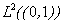employed in the numerical  experiments of Section 4 and in the numerical  experiments presented in . The wavelet mother functions have been obtained using the Symbolic Math Toolbox of MATLAB to implement the procedure described in .

In particular given the following parameters:

·      the minimum number M of vanishing moments that the wavelet basis considered must have,

·      the number N of subintervals of the interval (0,1) employed and the points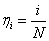, i=1,2,…,N-1,  where the subdivision of (0,1) in subintervals takes place,

the MATLAB code that constructs the specified wavelet basis can be easily written generalizing the programs reported here.

We begin showing some wavelet basis functions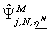, j=1,2,...,(N-1)M, obtained using as extra condition the regularity criterion (for more details and for the definition of the notation see ):

N0.m (download) J=M=1, N=2 and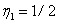;                                                     (1)
N1.m (downoald) J=M=2, N=2 and.                                                      (2)

The outputs of the programs N0.m, N1.m are the equations satisfied by the coefficients of the wavelet mother functions corresponding to the choice of the parameters specified in (1) and (2) respectively. The corresponding two non linear systems of equations have been solved with the command  solve of MATLAB. Let us note that all the wavelet  bases we present are uniquely determined up to a “sign permutation” (for more details about the meaning of “sign permutation” see ). One particular choice of this “sign permutation” leads to the following mother functions used in the numerical experience presented in Section 4:

Wavelet Basis 1: From (1) we obtain the mother function of the Haar’s basis: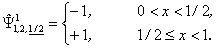Wavelet Basis 2: From (2) we obtain: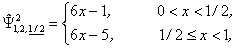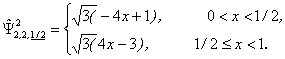Note that the function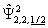is continuous in. The Wavelet Basis 2 is one of the multi-wavelets bases introduced by Alpert in .

Some wavelet basis functions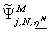, j=1,2,...,(N-1)M, obtained using as extra condition the “extra vanishing moments” criterion (for more details and for the definition of the notation see ), are the following ones:

superN0.m (download) J=3, M=1, N=4 and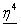=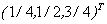,      (3)
J=6, M=2, N=4 and=.       (4)

The outputs of the programs superN0.m, superN1.m are the equations satisfied by the coefficients of the wavelet mother functions corresponding to the choice of the parameters specified in (3) and (4) respectively and a special choice of the request of “extra vanishing moments”. The corresponding two non linear systems of equations have been solved with the command solve of MATLAB. These wavelet bases are uniquely determined up to a “sign permutation” as well. One particular choice of this “sign permutation” leads to the following mother functions used in the numerical experience presented in Section 4:

Wavelet Basis 3: From (3) we obtain:Let us note that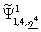and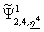have two vanishing moments while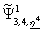has only one vanishing moment.

Wavelet Basis 4: From (4) we obtain:Let us note that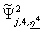, j=1,2,3,4,5, have three vanishing moments while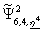has only two vanishing moments.

The choice of reporting in Section 4 and in  the numerical results obtained with the Wavelet Bases 1, 2, 3, 4 is motivated by the fact that these are the simplest bases among the bases introduced  in  that it is possible to construct and manipulate. Moreover the Bases 1 and 3 made of piecewise constant functions are particularly well suited to deal with piecewise constant functions. This type of functions is very important since it can be identified with digital signals and images.

Note that the direct and inverse wavelet transforms used in the numerical experience associated to these wavelet bases have  been implemented in FORTRAN 90.

4. Some numerical experiments: integral kernel sparsification, image compression and reconstruction

Integral kernel sparsification

In  we present some interesting properties of the wavelet bases introduced. In particular we show how the representation in these wavelet bases of certain classes of linear operators acting on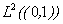may be viewed as a method for their “compression”, that is as a method to approximate them with sparse matrices.  In this way these operators can be applied to arbitrary functions belonging to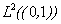in a “fast” manner and linear equations involving these operators can be approximated in high dimensional spaces and solved at an affordable computational cost. Moreover we show how the  orthogonality of the wavelets  to the  polynomials up to a given degree (the vanishing moments property) plays a crucial role in producing these sparse approximations. For more details see Theorems 4.1, 4.2 in .

In particular let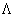be an integral operator  acting onof the form: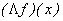=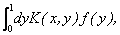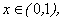f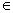,                                   (5)

where the kernel K is a real function of two variables. The compression algorithms we are interested in are based on the following observation. Whenis represented on  a wavelet basis, i.e. when the kernel K is expanded as a function of two variables on the wavelet basis, the calculation of the entries of the (infinite) matrix that represents the operator in the wavelet basis  involves the evaluation of some integrals. If the wavelet basis considered has several vanishing moments and we look at truncated wavelet expansions, that is to an expansion on a truncated wavelet basis, when the kernel K is sufficiently regular, thanks to the two estimates proven in the Theorems 4.1, 4.2 of , the fraction of the entries whose absolute value is smaller than a chosen threshold approaches one when the truncated expansion approaches the true expansion. The entries whose absolute value is smaller than a suitable threshold can be set to zero committing a “small” error. This property makes possible the approximation of the integral operator (5) with  sparse matrices and makes possible to solve numerically integral equations very efficiently.

Similar arguments can be made in the discrete case, where we consider  a piecewise constant  function defined on a bounded rectangular  domain  of the form: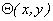=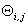,  (x,y)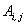, i,j=1,2,…,r,     (x,y)(0,1)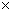(0,1),             (6)

where r is a positive integer and h>0 is such that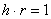and  whereis the “pixel” of indices i,j, i.e.=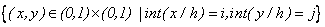, i,j=1,2,…,r. Note that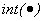denotes the integer part of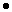.

The comparison between the original and the reconstructed “compressed” operator is made evaluating the relative difference between the original and the reconstructed compressed kernels in the Frobenius-norm and it is very satisfactory. We indicate with dim  the dimension of the vector space generated by the truncated wavelet basis and with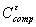the compression coefficient obtained with our algorithm when the truncation threshold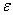>0 is used. The compression coefficientis defined as the ratio between dim2 and the number of matrix elements whose absolute value exceeds a threshold>0.

Each frame of the animations that follow shows four objects. Starting from the top left corner and moving in a clockwise sense we show:

·      the sparsity structure of the matrix obtained with the specified basis when the thresholdincreases. In particular the entries above the corresponding thresholdin absolute value are shown in black;

·      the compression coefficient;

·      the threshold;

·      the relative error in the Frobenius-norm made substituting the original operator with the reconstructed “compressed” operator after the truncation procedure as function of the threshold>0.

In  some tables comparing the compression coefficientsfor different values of the thresholdare shown.

Example 1.  In this example we consider the kernel: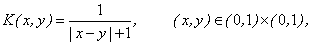and we expand it in the Wavelet Bases 2 and 4 generated by the mother functions reported in Section 3. We set to zero the entries of the resulting matrix whose absolute value is smaller than the threshold>0 and, whenincreases, we obtain the results shown in Animations 1 and 2.

Example 1: Sparsity structure of the matrix obtained with the Wavelet Basis 2 for dim=512.

Example 1: Sparsity structure of the matrix obtained with the Wavelet Basis 4 for dim=512.

Example 2.  In this example we compress the following piecewise constant function:(x,y),   i,j=1,2,…,dim, and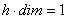,                  (x,y)(0,1)(0,1),

whereis the “pixel” of index i,j, defined previously. Remind that.  Animation 3 and 4 show some numerical results relative to Example 2 when dim=256 and we use the Wavelet Bases 1 and 3 generated by the mother functions reported in Section 3 respectively.

Example 2: Sparsity structure of the matrix obtained with the Wavelet Basis 1 for dim=256.

Example 2: Sparsity structure of the matrix obtained with the Wavelet Basis 3 for dim=256.

# Image compression and reconstruction

We  consider here some applications of the wavelet bases constructed in Section 3 in image compression and reconstruction. In particular we show some reconstructions of images and we  focus on  a lossy compression procedure. We limit our attention to grayscales images. Despite the appearance, compressing grayscale images is more difficult than compressing colour images. In fact human visual perception can distinguish more easily brightness (luminance) than colour (chrominance).

Going into details the steps in our image compression and reconstruction scheme are the following:

1. digitize the original image (i.e. transform the image in a matrix of numbers),
2. apply the wavelet transform  to represent the image through a set of wavelet coefficients,
3. manipulate (i.e. set to zero) some of the wavelet coefficients,
4. reconstruct the image with the inverse wavelet transform.

Note that the step 3 is the key step. In fact the lossy compression step is based on the fact that many of the wavelet coefficients are small in absolute value, so that they can be set to zero with little damage to the image. This procedure is called thresholding. In practice the most simple thresholding procedure consists in choosing a fixed tolerance and in doing the following truncation procedure: the wavelet coefficients whose absolute value falls below the tolerance are put to zero. Only the non zero wavelet coefficients after the thresholding procedure are used to build the so called compressed image. The goal is to introduce as many zeros as possible without losing a great amount of details. The crucial issue consists in the choice of  the threshold.

The following animations show three objects. In particular starting from the top left corner and moving in a clockwise sense we show:

·      the variation of the compressed images obtained with the specified wavelet basis according to the value of the threshold;

·      the compression coefficient;

·      the threshold;

To see only the behaviour of the compressed images please click on the right button.

In  some tables comparing the compression coefficientsfor different values of the thresholdare shown.

Example 3.  In this example we  consider the image of Figure 1 which is the famous Lena image. This image has 256x256 pixels.Figure 1. Lena: Original image

In Animation 5- 7 we show for different wavelet bases and several choices of the parameter dim  how the “compressed Lena image” improves when the thresholddecreases. Note that if all the wavelet coefficients are used in the  inverse wavelet transform (or equivalently,  if we take the threshold equal to zero) and an exact arithmetic is used the original image can be  reconstructed exactly.

Example 3: Compressed images with the Wavelet Basis 3 when dim=64 and the thresholddecreases.

Example 3: Compressed images with the Wavelet Basis 3 when dim=256 and the thresholddecreases

Example 3: Compressed images with the Wavelet Basis 4 when dim=512 and the thresholddecreases

Example 4.  We consider the image of Figure 2. In this image are evident three different types of objects: a shape (the black star), a number ('2025') and an inscription ('black'). This image has 278x268 pixels.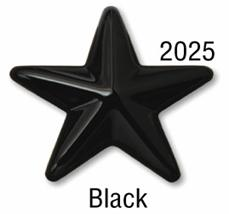Figure 2. Original image

The following Animations 8-9 show the compressed images of Figure 2 obtained for dim=128 and the Wavelet Bases 2, 4 when the thresholddecreases. Finally Animation 10 shows the compressed images of Figure 2 obtained for dim=256 and using the Wavelet Basis 3 when the thresholddecreases.

Example 4: Compressed images with the Wavelet Basis 2 when dim=128 and the thresholddecreases

Example 4: Compressed images with the Wavelet Basis 4 when dim=128 and the thresholddecreases

Example 4: Compressed images with the Wavelet Basis 3 when dim=256 and the thresholddecreases.

5. References

 B.K. Alpert, Wavelets and other bases for fast numerical linear algebra, in Wavelets: a tutorial in theory and applications, C. K. Chui, Editor, Academic Press, New York, (1992), pp. 181-216.

 B.K. Alpert, A class of bases in L2 for the sparse representation of integral operators, SIAM J. Math. Anal., 24(1) (1993), pp. 246-262.

 B.K. Alpert, G. Beylkin, R.R. Coifman, and V. Rokhlin, Wavelet-like bases for the fast solution of second-kind integral equations, SIAM J. Sci. Comp., 14 (1993), pp. 159-184.

 R.R. Coifman, Y. Meyer, Ondelettes et Opérateurs III: Opérateurs multilinéaires, Hermann, Paris, 1991.

 I. Daubechies, Ten Lectures on Wavelets, SIAM, Philadelphia, Pennsylvania, 1992.

 Daubechies, Orthonormal bases of compactly supported wavelets, Comm. Pure  Appl. Math, 41 (1998), pp. 909-996.

 L. Fatone,  M.C. Recchioni, and  F. Zirilli, New scattering problems and numerical methods in acoustics, in Recent Research Developments in Acoustics,  S.G.Pandalai Managing Editor, Transworld Research Network, Kerala, India,  2, 2005, pp. 39-69.

 L. Fatone, G. Rao,  M.C. Recchioni, and F. Zirilli, High performance algorithms based on a new wavelet expansion for time dependent acoustic obstacle scattering, Comm.  Comp. Phys. (2007), 2(6) (2007), pp. 1139-1173.

 L. Fatone,  M.C. Recchioni,  and F. Zirilli, A numerical method for time dependent acoustic scattering problems involving smart obstacles and incoming waves of small wavelengths, in   Mathematical Modeling of Wave Phenomena,  B.Nilsson, L.Fishman Editors, AIP  Conference Proceedings,  834 (2006), pp.108-121.

 L. Fatone,  M.C. Recchioni,  and F. Zirilli, Wavelet bases made of piecewise polynomial functions: approximation theory, quadrature rules and applications  to integral kernel sparsification and image compression, Applied Mathematics, 2 (2011) , pp.196-216.

 S. Mallat, Multiresolution approximation and wavelets, Trans.  Amer. Math.  Soc., 315 (1989), pp. 69-88.

 S. Mallat, Multifrequency channel decompositions of images and wavelet models,  IEEE Trans.  Acoust. Speech Signal Process.,  37 (1989), pp. 2091-2110 .

 Y. Meyer,  Ondelettes, fonctions splines et analyses graduées,  Rend. Sem. Mat. Univ. Politec. Torino, 45 (1988), pp.1-42.

 Y. Meyer, Ondelettes et Opérateurs I: Ondelettes,  Hermann, Paris, 1990.

 Y. Meyer, Ondelettes et Opérateurs II: Opérateurs de Calderón-Zygmund, Hermann, Paris, 1990.

 C.A. Micchelli and Y. Yu, Using the matrix refinement equation for the construction of wavelets on invariant sets, Appl. Comp. Harmonic Anal., 1 (1994), pp.391-401.

 C.A. Micchelli and Y. Yu, Reconstruction and decomposition algorithms for biorthogonal multiwavelets, Multidim. Syst. Sign. Process., 8 (1997), pp. 31-69.

### Informations request

We invite the user to fill up the following request of informations. We plan to use the informations acquired in this way for the following purposes:
1) improving the quality of this website and of the software,
2) enlarging our the knowledge and understanding of the problems of interest to the users of this website.